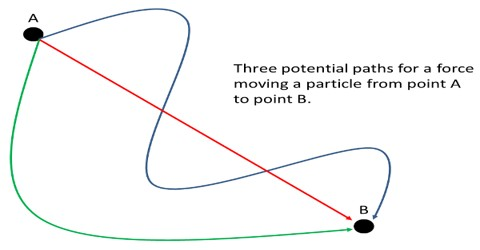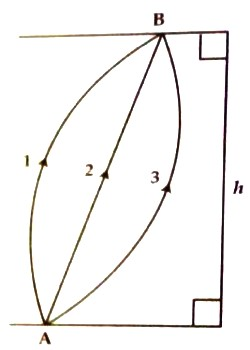# Conservative Force

Conservative Force

A force is said to be conservative if the work done by the force does not depend on the path along which the body moves, but depends only on the initial and final position. Work done by this force over a round trip is zero.

Examples: Gravitational force, electric force, restoring force of an ideal spring etc.

Characteristics of conservative force:

(1) This force depends only on position.

(2) Work done by the conservative force can completely be regained.

(3) This force does not depend on the path along which the body moves from one place to another place but depends only on the initial and the final positions of the body.

(4) Mechanical conservation principle of energy is followed due to the action of conservative force.Let an object of mass, ‘m’ be raised from a point A to a point B whose vertical height is h (Figure). The transfer of the object along the paths 1, 2 and 3 is different but gravitational force mg acting at any point in any path always acts vertically downward. The displacement of the object along the line of action of the gravitational force is ‘h’. So work done along each of the three paths is same and is given by,

W = – mgh.

Again, if the object is transferred from point A, following path 1, to point B and again transferred from B in initial position A, thus work done in the first case is,

W1 = – mgh [‘h’ is in opposite direction of g]

and in the second case,

W2 = mgh [‘h’in the same direction of g]

So, Total work done, W = W1 + W2 = – mgh + mgh = 0So, the gravitational force is conservative, work done by this force is recoverable. According to the characteristics of conservative force, a definition can be given of it. For example, a force is said to be conservative if the work done by the force does not depend on the path along which the body moves, but depends only on the initial and final position.